Over a million developers have joined DZone.{{announcement.body}}
{{announcement.title}}

# Classification From Scratch, Part 3 of 8: Logistics With Kernels

DZone 's Guide to

# Classification From Scratch, Part 3 of 8: Logistics With Kernels

### In this post, we'll be considering kernel-based techniques for using algorithms in R to conduct analyses on sets of data. Let's get to it!

· Big Data Zone ·
Free Resource

Comment (0)

Save
{{ articles.views | formatCount}} Views

This is the third post of our series on classification from scratch, following the previous post that introduced smoothing techniques, with (b)-splines. Consider here kernel-based techniques. Note that, here, we do not use the "logistic" model... it is purely non-parametric.

## Kernel-Based Estimated, From Scratch

I like kernels because they are somehow very intuitive. With GLMs, the goal is to estimate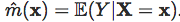Heuritically, we want to compute the (conditional) expected value on the neighborhood of X. If we consider some spatial model, where X is the location, we want the expected value of some variable Y, "in the neighborhood" of X. A natural approach is to use some administrative region (county, department, region, etc). This means that we have a partition of X (the space with the variable(s) lies). This will yield the regressogram, introduced in Tukey (1961). For convenience, assume some interval/rectangle/box type of partition. In the univariate case, consider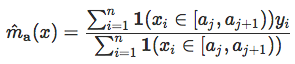or the moving regressogram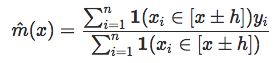In that case, the neighborhood is defined as the interval (x ± h). That's nice, but clearly very simplistic. If Xi= X and Xj=X-h+E (E>0), both observations are used to compute the conditional expected value. But if Xj=X-h-E, only Xi is considered. Even if the distance between Xj and Xj' is extremely, extremely small. Thus, a natural idea is to use weights that are a function of the distance between Xi's and X. Use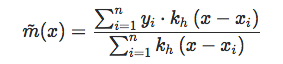where (classically)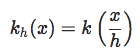for some kernel k (a non-negative function that integrates to one) and some bandwidth h. Usually, kernels are denoted with a capital letter K, but I prefer to use k, because it can be interpreted as the density of some random noise we add to all observations (independently).

Actually, one can derive that estimate by using kernel-based estimators of densities. Recall that: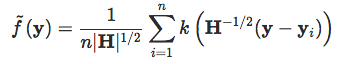Now, use the fact that the expected value can be defined as: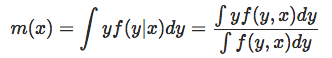Consider now a bivariate (product) kernel to estimate the joint density. The numerator is estimated by: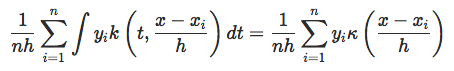While the denominator is estimated by: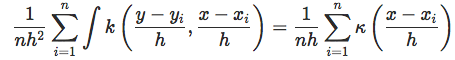In a general setting, we still use product kernels between Y and X and write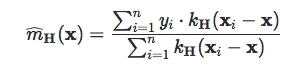for some symmetric positive definite bandwidth matrix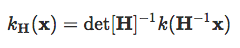Now that we know what kernel estimates are, let us use them. For instance, assume that k is the density of the N(0,1) distribution. At point x, with a bandwidth h we get the following code:

``````mean_x = function(x,bw){
w = dnorm((myocarde\$INSYS-x)/bw, mean=0,sd=1)
weighted.mean(myocarde\$PRONO,w)}
u = seq(5,55,length=201)
v = Vectorize(function(x) mean_x(x,3))(u)
plot(u,v,ylim=0:1,type="l",col="red")
points(myocarde\$INSYS,myocarde\$PRONO,pch=19)``````and of course, we can change the bandwidth.

``````v = Vectorize(function(x) mean_x(x,2))(u)
plot(u,v,ylim=0:1,type="l",col="red")
points(myocarde\$INSYS,myocarde\$PRONO,pch=19)``````We observe what we can read in any textbook: with a smaller bandwidth, we get more variance, less bias. "More variance," here, means more variability (since the neighborhood is smaller, there are fewer points to compute the average, and the estimate is more volatile), and "less bias" in the sense that the expected value is supposed to be computed at point x, so the smaller the neighborhood, the better.

## Using the ksmooth R Function

Actually, there is a function in R to compute this kernel regression.

``````reg = ksmooth(myocarde\$INSYS,myocarde\$PRONO,"normal",bandwidth = 2*exp(1))
plot(reg\$x,reg\$y,ylim=0:1,type="l",col="red",lwd=2,xlab="INSYS",ylab="")
points(myocarde\$INSYS,myocarde\$PRONO,pch=19)``````

We can replicate our previous estimate. Nevertheless, the output is not a function, but two series of vectors. That's nice to get a graph, but that's all we get. Furthermore, as we can see, the bandwidth is not exactly the same as the one we used before. I did not find any information online, so I tried to replicate the function we wrote before:``````g=function(bk=3){
reg = ksmooth(myocarde\$INSYS,myocarde\$PRONO,"normal",bandwidth = bk)
f=function(bm){
v = Vectorize(function(x) mean_x(x,bm))(reg\$x)
z=reg\$y-v
sum((z[!is.na(z)])^2)}
optim(bk,f)\$par}
x=seq(1,10,by=.1)
y=Vectorize(g)(x)
plot(x,y)
abline(0,exp(-1),col="red")
abline(0,.37,col="blue")``````There is a slope of 0.37, which is actually e-1. Coincidence? I don't know to be honest...

## Application in a Higher Dimension

Consider now our bivariate dataset, and consider some product of univariate (Gaussian) kernels.

``````u = seq(0,1,length=101)
p = function(x,y){
bw1 = .2; bw2 = .2
w = dnorm((df\$x1-x)/bw1, mean=0,sd=1)*
dnorm((df\$x2-y)/bw2, mean=0,sd=1)
weighted.mean(df\$y=="1",w)
}
v = outer(u,u,Vectorize(p))
image(u,u,v,col=clr10,breaks=(0:10)/10)
points(df\$x1,df\$x2,pch=19,cex=1.5,col="white")
points(df\$x1,df\$x2,pch=c(1,19)[1+(df\$y=="1")],cex=1.5)
contour(u,u,v,levels = .5,add=TRUE)``````

We get the following prediction:Here, the different colors are probabilities.

## k-nearest Neighbors

An alternative is to consider a neighborhood not defined using a distance to point X but the k-neighbors, with the n observations we got.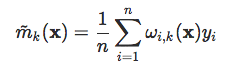where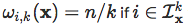with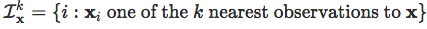The difficult part here is that we need a valid distance. If units are very different on each component, using the Euclidean distance will be meaningless. So, quite naturally, let us consider here the Mahalanobis distance.

``````Sigma = var(myocarde[,1:7])
Sigma_Inv = solve(Sigma)
d2_mahalanobis = function(x,y,Sinv){as.numeric(x-y)%*%Sinv%*%t(x-y)}
k_closest = function(i,k){
vect_dist = function(j) d2_mahalanobis(myocarde[i,1:7],myocarde[j,1:7],Sigma_Inv)
vect = Vectorize(vect_dist)((1:nrow(myocarde)))
which((rank(vect)))}``````

Here we have a function to find the k closest neighbor for some observation. Then two things can be done to get a prediction. The goal is to predict a class, so we can think of using a majority rule: the prediction for Yi is the same as the one the majority of the neighbors.

``````k_majority = function(k){
Y=rep(NA,nrow(myocarde))
for(i in 1:length(Y)) Y[i] = sort(myocarde\$PRONO[k_closest(i,k)])[(k+1)/2]
return(Y)}``````

But we can also compute the proportion of black points among the closest neighbors. It can actually be interpreted as the probability to be black (that's actually what was said at the beginning of this post, with kernels).

``````k_mean = function(k){
Y=rep(NA,nrow(myocarde))
for(i in 1:length(Y)) Y[i] = mean(myocarde\$PRONO[k_closest(i,k)])
return(Y)}``````

We can see on our dataset the observation, the prediction based on the majority rule, and the proportion of dead individuals among the 7 closest neighbors.

``````cbind(OBSERVED=myocarde\$PRONO,
MAJORITY=k_majority(7),PROPORTION=k_mean(7))
OBSERVED MAJORITY PROPORTION
[1,]        1        1  0.7142857
[2,]        0        1  0.5714286
[3,]        0        0  0.1428571
[4,]        1        1  0.5714286
[5,]        0        1  0.7142857
[6,]        0        0  0.2857143
[7,]        1        1  0.7142857
[8,]        1        0  0.4285714
[9,]        1        1  0.7142857
[10,]        1        1  0.8571429
[11,]        1        1  1.0000000
[12,]        1        1  1.0000000``````

Here, we got a prediction for an observed point, located at Xi, but, actually, it is possible to seek the k closest neighbors of any point X. Back on our univariate example (to get a graph), we have:

``````mean_x = function(x,k=9){
w = rank(abs(myocarde\$INSYS-x),ties.method ="random")
mean(myocarde\$PRONO[which(w&lt;=9)])}
u=seq(5,55,length=201)
v=Vectorize(function(x) mean_x(x,3))(u)
plot(u,v,ylim=0:1,type="l",col="red",lwd=2,xlab="INSYS",ylab="")
points(myocarde\$INSYS,myocarde\$PRONO,pch=19)``````That's not very smooth, but we do not have a lot of points either.

If we use that technique on our two-dimensional dataset, we obtain the following:

``````Sigma_Inv = solve(var(df[,c("x1","x2")]))
u = seq(0,1,length=51)
p = function(x,y){
k = 6
vect_dist = function(j)  d2_mahalanobis(c(x,y),df[j,c("x1","x2")],Sigma_Inv)
vect = Vectorize(vect_dist)(1:nrow(df))
idx  = which(rank(vect)&lt;=k)
return(mean((df\$y==1)[idx]))}
v = outer(u,u,Vectorize(p))
image(u,u,v,xlab="Variable 1",ylab="Variable 2",col=clr10,breaks=(0:10)/10)
points(df\$x1,df\$x2,pch=19,cex=1.5,col="white")
points(df\$x1,df\$x2,pch=c(1,19)[1+z],cex=1.5)
contour(u,u,v,levels = .5,add=TRUE)``````This is the idea of local inference, using either kernel on a neighborhood of X or simply using the k nearest neighbors. Next time, we will investigate penalized logistic regressions.

Topics:
big data ,kernel ,data analytics ,logistic regression

Comment (0)

Save
{{ articles.views | formatCount}} Views

Published at DZone with permission of

Opinions expressed by DZone contributors are their own.

# {{ parent.title || parent.header.title}}

{{ parent.tldr }}

{{ parent.urlSource.name }}